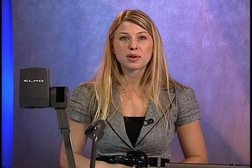PLAY PREVIEW

### Segments in this Video

#### Outline: T-Tests: Lecture 7(01:45)

FREE PREVIEW

In this lecture, Prof. Naomi Lowe explains T-tests including one-sample, dependent/paired, standard deviation and variance, degrees of freedom, and T distribution.

#### Intro to Student's T: SD/Var of a Sample(07:35)

William Gosset published articles under the alias "Student" while working for Guinness. Use Student's T when the population variance is unknown. Standard deviation is the square root of variance; see an unbiased estimate example.

#### Intro to Student's T: Degrees of Freedom (DF)(04:59)

DF is the number of scores that are free to vary. Learn the formula for calculating variance using degrees of freedom and see examples.

#### Intro to Student's T: Variance of Means(04:02)

This formula determines the spread of all possible sample means. See an example of the standard deviation of the distribution of means.

#### Intro to Student's T: T Distribution(05:22)

The distribution of all possible sample means depends on the number in the sample. The T-curve extends with smaller degrees of freedom on the graph.

#### One-Sample T(12:44)

The T-test works with a sample from one population. This example determines the knowledge of individuals who have seen paid political ads using a T formula that is similar to the Z formula.

#### Dependent/Paired T(12:57)

The T-test uses one sample two times or paired samples. Use the difference scores, assume the population mean difference is null, and use the same T-distribution to make the comparison. See examples of a dependent T-test and a paired T-test.

#### Power & Effect Size(06:06)

Insert the effect size into a power table to determine possible powers. See an effect size example for one-sample T-tests and dependent T-tests.

For additional digital leasing and purchase options contact a media consultant at 800-257-5126
(press option 3) or sales@films.com.

# T-Tests: Lecture 7

Part of the Series : Statistics With Naomi Lowe
 DVD (Chaptered) Price: \$129.95 DVD + 3-Year Streaming Price: \$194.93 3-Year Streaming Price: \$129.95

Share

### Description

In this video lecture, Professor Naomi Lowe provides an introduction to T-Tests.

Length: 56 minutes

Item#: BVL150107

ISBN: 978-1-64347-559-2Closed Captioned